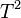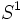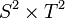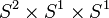# Product of 2-sphere and 2-torus

## Definition

This topological space is defined as the Cartesian product, equipped with the product topology, of the 2-sphere$S^2$ and the 2-torus$T^2$ (which itself is the product of two copies of the circle$S^1$). It is denoted$S^2 \times T^2$ or$T^2 \times S^2$ or$S^2 \times S^1 \times S^1$ or$S^1 \times S^1 \times S^2$.

## Topological space properties

Property Satisfied? Is the property a homotopy-invariant property of topological spaces? Explanation Corollary properties satisfied/dissatisfied
manifold Yes No product of manifolds is manifold satisfies: metrizable space, second-countable space, and all the separation axioms down from perfectly normal space and monotonically normal space, including normal, completely regular, regular, Hausdorff, etc.
path-connected space Yes Yes path-connectedness is product-closed satisfies: connected space, connected manifold, homogeneous space (via connected manifold, see connected manifold implies homogeneous)
simply connected space No Yes Product of multiple spaces, one of which (the circle) is not simply connected. dissatisfies: simply connected manifold
rationally acyclic space No Yes Product of spaces neither of which is rationally acyclic. dissatisfies: acyclic space, weakly contractible space, contractible space
space with Euler characteristic zero Yes Yes Product of two spaces, one of which (the 2-torus) has Euler characteristic zero. Note that Euler characteristic of product is product of Euler characteristics
space with Euler characteristic one No Yes See above, the Euler characteristic is zero
compact space Yes No Product of compact spaces, see Tychonoff's theorem satisfies: compact manifold, compact polyhedron, polyhedron (via compact manifold), compact Hausdorff space, and all properties weaker than compactness